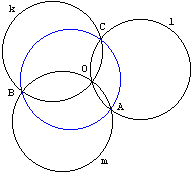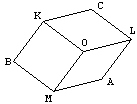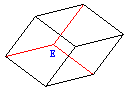# Three equal circles

Here is the problem:If three circles having the same radius pass through a point, the circle through their other three points of intersection also has the same radius.

The circles are denoted k, l, m. The points of their intersection are 0, A, B, C.

SolutionIf three circles having the same radius pass through a point, the circle through their other three points of intersection also has the same radius.## Solution

 The picture above is somewhat incomplete. We are dealing with circles after all. A circle is defined by its center and its radius. In the original picture the center points are missing. Let denote them K, L, M. Now it also might be important to indicate what distances are equal. For this is our data: the three circles all have the same radius.The nine equal segments appear to form a well known shape. It's a projection of a parallelepiped on a plane.Normally we would draw such a projection with three more edges that were hidden above. Now we have 12 equal segments. The newly drawn edges meet at a previously hidden vertex E which is equidistant from points A, B, and C.(A formal counterpart of the above argument appears elsewhere. Also, there is a very interesting circumstance where the above configuration emerges naturally but still unexpectedly.)

## References

1. R. Honsberger, Mathematical Gems II, MAA, 1976, pp. 20-21
2. G. Polya, Mathematical Discovery, John Wiley and Sons, 1981, Ch. 10.### 2D Problems That Benefit from a 3D Outlook### 3 Equal and Concurrent Circles